# Electronics and Communication Engineering - Signals and Systems

Exercise : Signals and Systems - Section 1
36.
The signal defined by the equations f(t) = 0 for t < 0, f(t) = E for 0 ≤ ta and f(t) = 0 for t > a is
a step function
a pulse function
a shifted step function originating at t = a
none of the above
Explanation:

It is a pulse lasting for t = a.

37.
Inverse Laplace transform of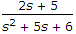is
2 exp (- 2.5 t) cosh (0.5 t)
exp (- 2 t) + exp (- 3 t)
2 exp (- 2.5 t) sinh (0.5 t)
2 exp (- 2.5 t) cos 0.5 t
Explanation: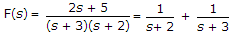f(t) = e-2t + e-3t.

38.
Two function g1(t) and g2(t) with correlation of 6 has average power of 4 and 5 respectively. The power of g1(t) + g2(t) is
9
21
3
15
Explanation:

Power of two correlated signal is P = P1 + P2 +2R1, 2(t).

39.
A box has 4 white and 3 red balls. Two balls are taken out in succession. What is the probability that both are white?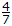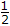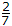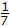The probability that first ball is white is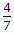and the probability the second ball is white is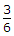. The probability that both are white is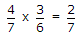.